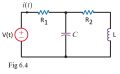# Determining capacitance for an in phase Voltage & Current

#### LegendIV

Joined Oct 16, 2018
1Determine the value of capacitor C to ensure the current is in phase with the voltage given:
V(t) = 60*e^j(250t + 30°)
R1 = 8 ohm
R2 = 22 ohm
L = 10 + (2*R2)mH

what is the capacitance?

Please note that this is not a homework assignment but I really need to understand it for an upcoming exam.

I know that to get current in phase with voltage, we can't have an imaginary part for our impedance, i.e purely resistive circuit. This also means that the inductor & capacitor will even out creating infinite impedance (i think). I am unable to calculate the answer.

(NOTE: the correct answer is 81.05 uF)

Joined Mar 10, 2018
4,057
I think the general approach is observe that the voltage source has a
phase shift of +30 degrees. Therefore the impedance must also present
as 30 degrees at radian freq of 250t.

So solve the network Z presented to V source, convert to rectangular form
(Z(jw)), then express phase angle of Z and solve network C to present 30 degrees.

I would have to break out the textbooks to do this myself, 50+ years since last time
I knew the difference between 1 and 2.......

Regards, Dana.

#### noweare

Joined Jun 30, 2017
113
I could not compute the correct answer which is 81.05 uf. I did verify the answer using LT Spice. This one is harder than it looks. I think the math gets a bit messy. The impedance would need to be resistive only since the current & voltage are in phase with each other.

#### fernandopv

Joined Oct 20, 2018
10
In this case is more direct to consider that C || (R2+XL) must be purely resistive.
This way :

jwC + 1/( R2 + jwL) must have its imaginary part equal to zero.

Then, C == L/(R2^2 + (wL)^2) giving the correct result.

#### noweare

Joined Jun 30, 2017
113
Last edited:

#### Jony130

Joined Feb 17, 2009
5,176
Is that maximum that your using to do the math?
In this case it is a Mathematica software .

#### The Electrician

Joined Oct 9, 2007
2,778
In this case is more direct to consider that C || (R2+XL) must be purely resistive.
This way :

jwC + 1/( R2 + jwL) must have its imaginary part equal to zero.

Then, C == L/(R2^2 + (wL)^2) giving the correct result.
It was appropriate to show all the details for the general case given the apparent skill level of the TS. For example, suppose R1 is replaced by a 10 mH inductor labeled L1. It's not so easy now, but the procedure I showed will get the result.

•cmartinez

#### fernandopv

Joined Oct 20, 2018
10
It was appropriate to show all the details for the general case given the apparent skill level of the TS. For example, suppose R1 is replaced by a 10 mH inductor labeled L1. It's not so easy now, but the procedure I showed will get the result.
Sure, but longuer enough to try to avoid it an exam. After all, why do you think a teacher give this specific circuit for? Perhaps he wants to know if somebody understads the physics appart of applying correctly the algebra.

#### The Electrician

Joined Oct 9, 2007
2,778
Sure, but longuer enough to try to avoid it an exam. After all, why do you think a teacher give this specific circuit for? Perhaps he wants to know if somebody understads the physics appart of applying correctly the algebra.
If it was given as part of a physics course, then perhaps the instructor is testing the student's knowledge of the physics. If it's from an EE course, I would think testing the understanding of phasor algebra would the goal, the physics having been learned earlier.

Understanding the general principles of phasor analysis must come first; then learning to recognize shortcuts will surely follow.

#### noweare

Joined Jun 30, 2017
113
Good job coming up with this Electrician. Honestly I couldn't see how any teacher would give such a number crunching problem like this on a test. If your supposed to solve this with pencil & paper, good luck.

#### The Electrician

Joined Oct 9, 2007
2,778
Good job coming up with this Electrician. Honestly I couldn't see how any teacher would give such a number crunching problem like this on a test. If your supposed to solve this with pencil & paper, good luck.
You raise an interesting issue. I'm an old guy and I remember when calculators first replaced slide rules. Teachers were worried that students wouldn't learn to do the arithmetic themselves--they would just use their calculator. It seemed to me that this wouldn't be a problem if the teachers dealt with the situation properly. The solution, as it seemed to me, was this: just give harder problems, problems that would need a calculator to solve in a reasonable time frame.

The problem in this thread can be solved by considering the components as admittances rather than as impedances as fernandopv did in post #5, and further realizing that R1 in series cannot affect whether the imaginary part of Z is zero or not, so R1 need not be considered at all. This can be done by hand if the student has been practicing complex algebra. But if the problem were very much more complicated, for example if R1 were an inductor, the harder problem could be assigned in a classroom setting where the students can use their laptop or a calculator to help.

•cmartinez

#### cmartinez

Joined Jan 17, 2007
7,022
the harder problem could be assigned in a classroom setting where the students can use their laptop or a calculator to help.
•